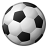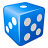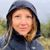# select random points

7066
7
01-30-2014 07:19 PMNew Contributor
Hey all!

I have sounding data with tens of thousands of points per feature. I need to reduce the points significantly in density, and was hoping there was a way to randomly select points through an arcmap tool. While the create random points tool does what I need, it does not seem to maintain the attribute data (eg. depth) that I need with each point. Any suggestions?
Tags (3)
7 Repliesby
Occasional Contributor II
I think the Create Random Points tool does just that: creates new points randomly, rather than selecting existing points randomly.

You could try adding a new field to your existing points, then calculating a random 1 or 0 for each point, then just select out the 1s. I think it could still be a bit random how many 1s you get though, it might not reduce the points by much.

If there was a certain factor you wanted to reduce the data by, and as long as the OBJECT_IDs aren't sorted in any way, you could calculate a modulo in your field, e.g. to just pick out every 10th point, you'd calculate OBJECT_ID % 10 (which would give you the remainder left if you divided the OBJECT_ID by 10) and select all the 0s in that field.

Hope that makes sense, if not let me know and I will try to explain better!

DanbyEsri Regular Contributor
Subset Features is designed to do that.Occasional Contributor IIIOccasional Contributor III
Subset Features is designed to do that.

*** Requires Geostatistical Analyst ***Occasional Contributor III
This code will populate an existing attribute with a random number.  Run the script.  Then, for example, select values less than 0.2 to select about 20% of your points.

```#Populates an existing attribute with a randomly generated value

try:
import operator, os, random, traceback, sys, arcpy

#Path to the feature class.
fc = arcpy.GetParameterAsText(0)
#The Attribute to populate...
attribute = arcpy.GetParameterAsText(1)

uc = arcpy.UpdateCursor(fc)
for row in uc:
x = random.random()
row.setValue(attribute, x)
uc.updateRow(row)

except arcpy.ExecuteError:
msgs = arcpy.GetMessages(2)
except:
tb = sys.exc_info()
tbinfo = traceback.format_tb(tb)
pymsg = "PYTHON ERRORS:\nTraceback info:\n" + tbinfo + "\nError Info:\n" + str(sys.exc_info())
msgs = "ArcPy ERRORS:\n" + arcpy.GetMessages(2) + "\n"